## Example Questions

### Example Question #5 : How To Find The Length Of The Diagonal Of A Rectangle

A rectangle has a height ofand a base of. What is the length of its diagonal rounded to the nearest tenth?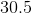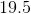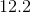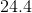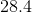Explanation:

1. Use Pythagorean Theorem with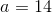and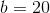.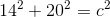2. Solve for, the length of the diagonal: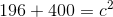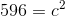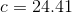This rounds down tobecause the hundredth's place () is less than.

### Example Question #6 : How To Find The Length Of The Diagonal Of A Rectangle

The sides of rectangle ABCD are 4 in and 13 in.

How long is the diagonal of rectangle ABCD?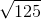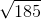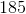Explanation:

A diagonal of a rectangle cuts the rectangle into 2 right triangles with sides equal to the sides of the rectangle and with a hypotenuse that is the diagonal. All you need to do is use the pythagorean theorem:where a and b are the sides of the rectangle and c is the length of the diagonal.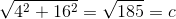### Example Question #1 : How To Find The Length Of The Diagonal Of A Rectangle

A power company needs to run a piece of wire across a rectangular plot of land and must do so diagonally.  The land is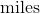byin measurement. If it costs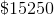for each mile of wire deployed, how much is the expected cost of this project?  Round to the nearest cent.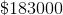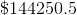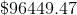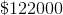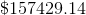Explanation:

Notice that this problem could be represented as follows: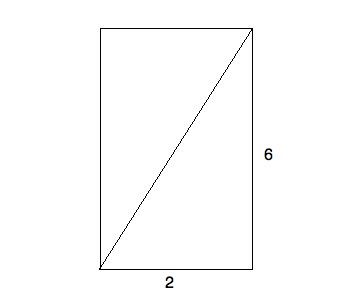This means that you can find the distance of the wire merely by using the Pythagorean theorem: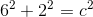Solving for, you get: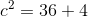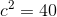Thus,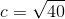Using your calculator, multiply this by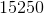.  This gives you approximately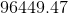dollars in expenses.

### Example Question #2 : How To Find The Length Of The Diagonal Of A Rectangle

What is the diagonal of a rectangle with sides of lengthand? Round to the nearest hundredth.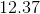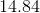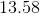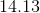Explanation:

You could draw this rectangle as follows: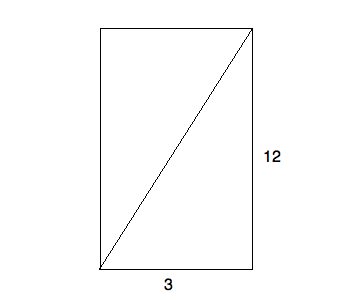Solving for the diagonal merely requires using the Pythagorean theorem. Thus, you know: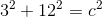or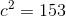, meaning that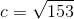This is approximately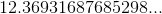Thus, the answer is.

### Example Question #3 : How To Find The Length Of The Diagonal Of A Rectangle

What is the area of a rectangle with a diagonal of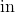and one side that is?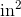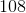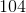Explanation:

Based on the description offered in the question, you know that your rectangle must look something like this: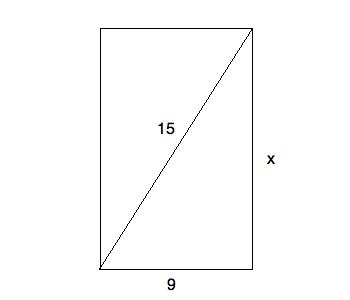Using the Pythagorean theorem, you can solve for the unknown side: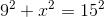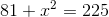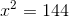Thus,is.  This means that the area is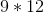or.

### Example Question #4 : How To Find The Length Of The Diagonal Of A Rectangle

Mark bought a TV at the store that was listed as 36in x 24in. He needs to figure out the diagonal to make sure the television is large enough but he left his measuring tape at his mother's house. What is the diagonal length of this television's screen in terms of inches?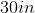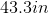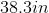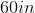Explanation:

Finding the diagonal of a rectangle is essentially a problem with triangles. If we set up a right triangle with legs 24 in. and 36 in., and setto be the diagonal (the hypotenuse), we can use the Pythagorean Theorem to solve for: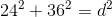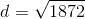This comes out to be about 43.3 in.